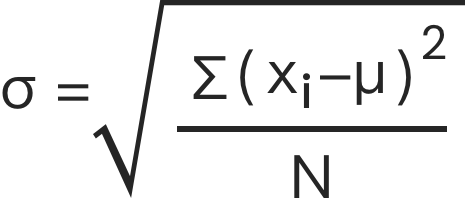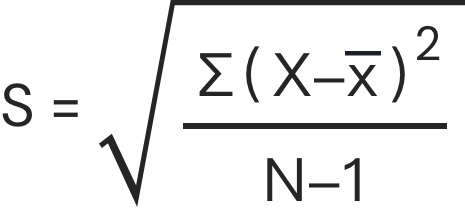### Still have math questions?

Scientific Notation Length Conversion Area Conversion

### Calculator

Population
Sampleσ = population standard deviation
N = the size of the population
xi = each value from the population
μ = the population meanσ =

#### Knowledge

Standard deviation is a measure of the amount of variation or dispersion of a set of values.A low standard deviation indicates that the values tend to be close to the mean (also called the expected value) of the set, while a high standard deviation indicates that the values are spread out over a wider range.

Standard deviation may be abbreviated SD, and is most commonly represented in mathematical texts and equations by the lower case Greek letter sigma σ, for the population standard deviation, or the Latin letter s, for the sample standard deviation.σ = population standard deviation
N = the size of the population
xi = each value from the population
μ = the population means = sample standard deviation
∑ = sum of…
X = each value
x = sample mean
n = number of values in the sample

#### Example 1

Population standard deviation of grades of eight students.

Suppose that the entire population of interest is eight students in a particular class. For a finite set of numbers, the population standard deviation is found by taking the square root of the average of the squared deviations of the values subtracted from their average value. The marks of a class of eight students (that is, a statistical population) are the following eight values: (2,4,4,4,5,5,7,9).

These eight data points have the mean (average) of 5:

μ = 2+4+4+4+5+5+7+9 8 = 40 8 = 5.

First, calculate the deviations of each data point from the mean, and square the result of each:

(2-5)2 = (-3)2 = 9

(4-5)2 = (-1)2 = 1

(4-5)2 = (-1)2 = 1

(4-5)2 = (-1)2 = 1

(5-5)2 = 02 = 0

(5-5)2 = 02 = 0

(7-5)2 = 22 = 4

(9-5)2 = 42 = 16

The variance is the mean of these values:

σ2 = 9+1+1+1+0+0+4+16 8 = 32 8 = 4.

and the population standard deviation is equal to the square root of the variance:

σ = 4 = 2.

#### Example 2

A sample of 444 students was taken to see how many pencils they were carrying.

Calculate the sample standard deviation of their responses: 2, 2, 5, 7.

First, find the mean.

x = 2+2+5+74 = 164 = 4

Second, subtract the mean from each score and square each deviation.

(2-4)2 = (-2)2 = 4

(2-4)2 = (-2)2 = 4

(5-4)2 = (1)2 = 1

(7-4)2 = (3)2 = 9

And divide the sum by one less than the number of data points then take the square root of the result.

4+4+1+9 = 18

184-1 = 183 = 6

s = 6 ≈ 2.45

Please provide numbers only. Please provide at least two numbers. Please provide 2 to 10 non-zero integer numbers. None